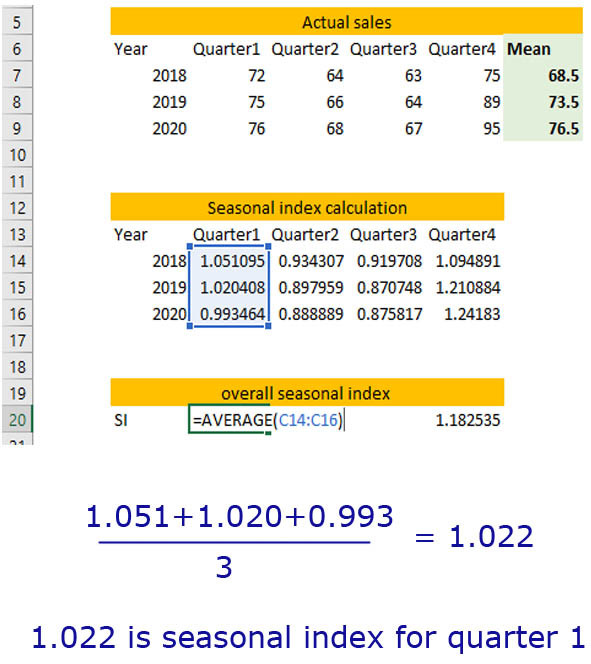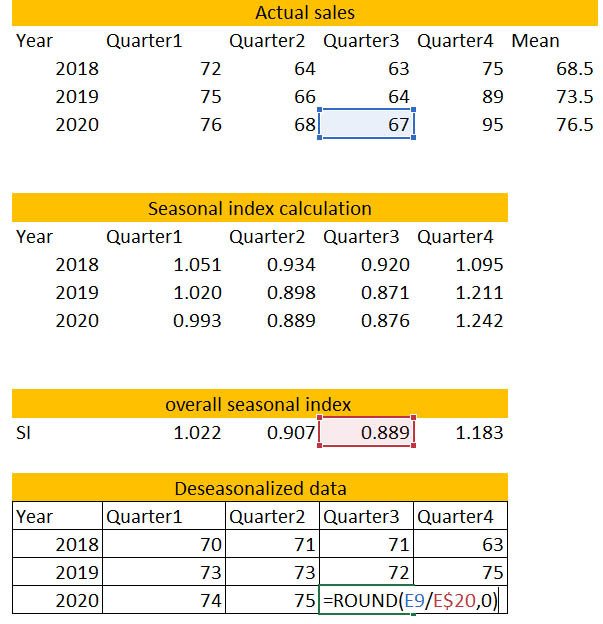Search
• Marek Vavrovic

# Seasonal Indices (Seasonal Index)

When data under analysis has a seasonal influence on it, further investigation cannot continue without deseasonalising the data. This involves calculating seasonal indices to tell us how a particular season (day/month/quarter) compares to the average season.

Interpreting seasonal indices

Seasonal indices have an average value of 1. This can be converted into a percentage for easier interpretation. A seasonal index of 1.3 (or 130%) would indicate that that season had 30% more than the seasonal average. An example is where Christopher works all throughout the year at a ice-cream shop and earns an average of \$100,000 a season for it. If the seasonal index for summer was 1.5, then that means Christopher earns 50% more than the average \$100,000. Likewise, a seasonal index of 0.6 in winter would indicate that Christopher earns 40% less than the seasonal average.

Seasonal Index is a measure of how a particular season through some cycle compares with the average season of that cycle. By deseasonaling the data, we are removing the seasonal fluctuation, or patterns in the data to predict or approximate future data values.

Suitable for:

- Data with seasonal variation only

- not for trends and seasonality in the same data set

- short term forecasting

ExampleCalculate the seasonal index of quarterly sales (\$ in thousands) for the data given below.

step 1: Calculate the yearly average. Find average for the given year.

For example 2018=( 72+64+63+75) / 4 = 68.5step 2: Work out the sales figures as a proportion of the yearly average. Divide the actual sales amount for the given quarter by its yearly average (mean).If you have just one year of data (for instance just year 2018) these numbers would be your seasonal index. But we have more than one year of data therefore there is one more step involved in order to get the correct seasonal index value.

step 3 : Now when I have the yearly proportions (seasonal indexes) for all 3 years of data, I can work out an overall seasonal index for each of these quarters by calculating average by quarters.A handy rule to know is that the Seasonal Indices will always add up to the number of time periods you have. If you are dealing with quarters your seasonal index will add up to 4. If it is month and there are 12 of them your seasonal indices will add up to 12. You can also use the days of week, there are 7 of them.Step 4: Once you know what the seasonal indexes are, you can then de-seasonalize the data. What the de-seasoning does is, it smoothes it out. It takes away some of the seasonal fluctuation that occurs.DE-seasonalized value = actual sale / seasonal index

Round it to the next whole number.I want to compare the seasonal data with deseasonal data. Take them into power query and use

Unpivot Other Columns transformation step.When we plot these two sets of time series data on the same axis, we can see what deseasonalised series is doing for us. The actual data has a big fluctuation, you can see the peaks and troughs because it is a seasonal data. The DS values are much flatter or straighter because we have taken out the fluctuation. So, we can see what is really happening with these sales figures.

You can also see the trend line which is telling us, that the sale is over time increasing slightly. It has a slight positive trend what is good for sales.2018_Q4: deseasonalised value was actually very low according to the overall trend.

2018_Q4: actual sale value, according to that point it looks like the sale were up about that time but because that’s the end of the year, that’s maybe a Christmas sale. If you have a look on the Christmas sales for 2019 and 2020, are much higher. So, in 2018_Q4 they had quite low sale deseasonalised. That’s the kind of stuff the deseasonalised data can tell us.Example 2

Determine the equation of the least squares trend line for the deseasonalised data, and calculate the deseasonalised sales prediction for the first quarter of 2021 .

2018_Q1 =1, 2018_Q2 = 2, 2018_Q3=3,2018_Q4=4, 2019_Q1=5,2019_Q2=6... first quarter of 2021: x=13. Excel has generate the least square regression for me: y=0.818x + 67.348. We can use this equation to figure out the sale for the first quarter of 2021. (Round to whole number)Example 3

Using this deseasonalised sales prediction, estimate the actual sales during the first quarter of 2021 .

The deseasonalised prediction was calculated as y=0,818x + 67,348 = 77,98. To calculate the prediction for the actual sale we are going to need the Seasonal Index for quarter 1 which is 1,022. If you remember the equation for deseasonalised value = actual / seasonal index.

We computed the deseasonalised value in the previous example using the least square equation.

Actual = Deseasonalised * Seasonal Index

Actual = \$80.000 (I rounded to whole number)## Recent Posts

See All

#### Index

Information functions Filter functions Table manipulation functions Grouping and summarizing Time intelligence functions OPENINGBALANCEYEAR OPENINGBALANCEYEAR(<Expression>,<Dates> [,<Filter>][,<YearEn